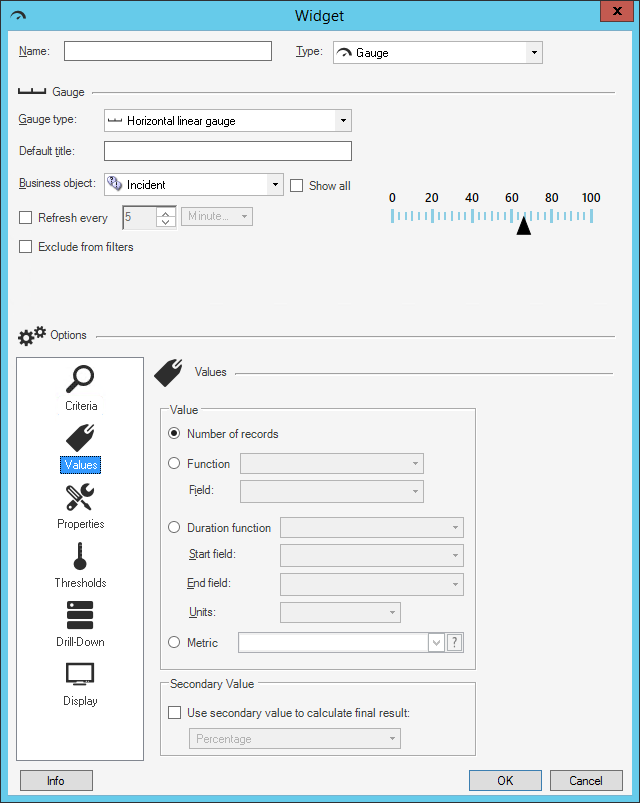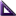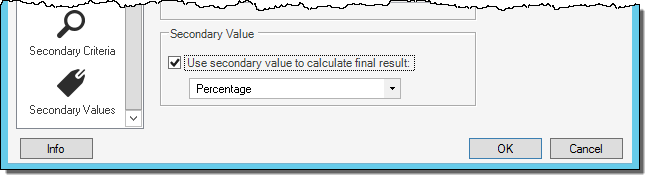# Define a Value for a Gauge Widget

Use the Values page (accessed within the Gauge Widget Properties window) to define the value to display in a Gauge Widget. Values can be based on:

• Number of records.
• Function: Calculated average, maximum, minimum, or total (ex: Average customer satisfaction rating).
• Duration: Calculated average, maximum, minimum, or total based on an amount of time (ex: Average amount of time that Incidents take to resolve each day).
• Metric: Metric value, either simple Query or calculated.
• Secondary value: Value calculated using a secondary value (ex: What percentage of Incidents are P1 (P1 Incidents/All Incidents x 100 = WHAT%)). If you had 70 Incidents and 43 were closed, your P1 percentage would be 61.4%.

The Gauge Widget Properties window is accessed from within the Widget Manager when you create or edit a Gauge Widget.

Good to know:

• A Metric is a reusable measurement query that can be used in place of the other calculated values (ex: Function, Duration, Calculated value). For more information, refer to the Metrics documentation.

To define a value for a Gauge Widget:

1. Create a Widget
2. In the Type drop-down, select Gauge.
3. Click the Values page.4. Define the value to display in the gauge. A value can be:
• Number of records: Select this option to display the number of records that match the criteria (ex: 15 Open Incidents).
• Function: Select this option to display a value that calculates an average, maximum, minimum, or total value based on a selected Field, and then select the function and Field (ex: Average customer satisfaction rating).
• Duration Function: Select this option to display a value that is calculated based on an amount of time (ex: Average amount of time that Incidents take to resolve each day), and then select the Start/End Fields and the units (duration).

Example: Display the average amount of time that Incidents take to resolve each day (ex: Start Field = Created Date/Time, End Field = Closed Date/Time, and Units = Days).

A percent gauge is calculated by [Value]/[Secondary Value] * 100. If you are defining a percent gauge, define the numerator (value) here and the denominator (secondary value) in the next step. For example, for the calculation P1 Incidents/All Incidents x 100 = WHAT%, define P1 Incidents here.

• Metric: Select this option to use a Metric value, and then select an existing CSMMetric in the drop-down; or, click the Metric button to launch the Metric Manager, and then create a Metric.

If you select a Metric, the Metric Properties window pops up, prompting you to select which value you would like to retrieve for use in the gauge. The value can be retrieved from the local cache or from the database. You can also access the Metric Properties window by pressing the Metric icon. For more information about Metrics and caching, refer to the Metrics documentation.

5. (Optional) Define a secondary value to use for a calculation:

The calculation for a percentage is (Criteria) / (Secondary Criteria) * 100. If you want a percentage of Open Incidents out of all Incidents, your Criteria would be Open Incidents and your Secondary Criteria would be All Incidents.

1. Use Secondary Value to Calculate Final Result: Select this check box to display a calculated value on the Widget, and then define how to calculate the value by selecting an option in the drop-down (currently, percentage is the only option).

The Secondary Criteria and Secondary Values pages appear in the Options list.2. Define the secondary criteria/value to use in the calculation.
6. Select OK.